• ## 传递函数

万次阅读 2018-07-07 19:08:55
一、传递函数与微分方程是一一对应的关系 传递函数、频率特性、频域分析与时域的各个环节是一一对应的，好处在于便于分析计算。 频域本身没有什么物理意义，它的意义就在于与时域环节一 一对应，见到频域的传递...


一、传递函数与微分方程是一一对应的关系

传递函数、频率特性、频域分析与时域的各个环节是一一对应的，好处在于便于分析计算。

频域本身没有什么物理意义，它的意义就在于与时域环节一 一对应，见到频域的传递函数，就想到它对应的时域的环节。

以惯性环节为例，看到传递函数K/（Ts+1）就想到惯性环节的过程是先对输入进行积分，等到3~4T的时候才保持恒值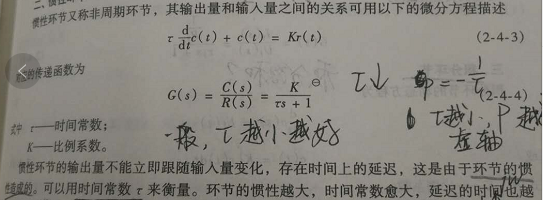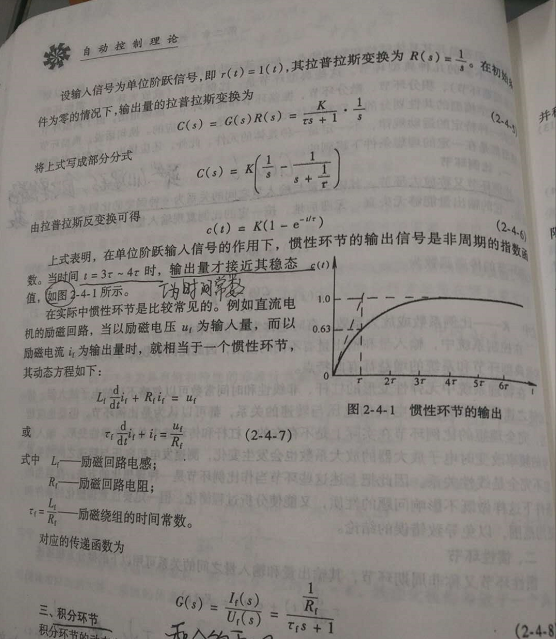确实有点惯性的意思，给它一个输入，它不愿意马上就变过去，还想保持原来的状态，于是就扭扭捏捏地变过去。二、惯性环节

惯性环节的输出一开始并不与输入同步按比例变化，直到过渡过程结束,y(t)才能与x(t)保持比例。这就是惯性地反映。惯性环节的时间常数就是惯性大小的量度。凡是具有惯性环节特性的实际系统，都具有一个存储元件或称容量元件，进行物质或能量的存储，如电容、热容等。由于系统的阻力，流入或流出存储元件的物质或能量不可能为无穷大，存储量的变化必须经过一段时间才能完成，这就是惯性存在的原因。

eg.电机就是惯性环节：给它施加电压，不能马上转到电压对应的速度；撤去电压，Motor不能马上停。

惯性环节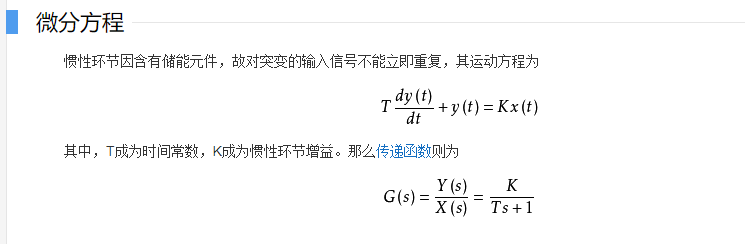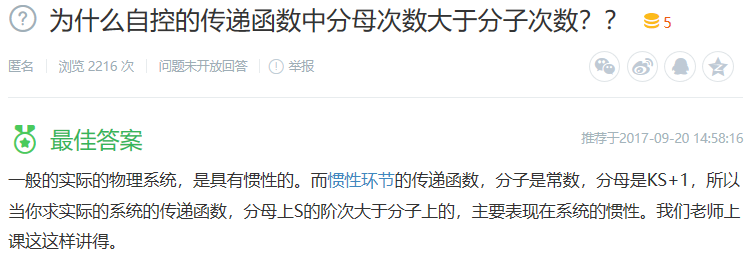分母阶次大于分子阶次，主要表现在系统的惯性。

这句话值得思考，像上面的一阶惯性环节，分母阶次比分子大一阶，其实就是多了一阶导函数。

三、惯性环节与积分环节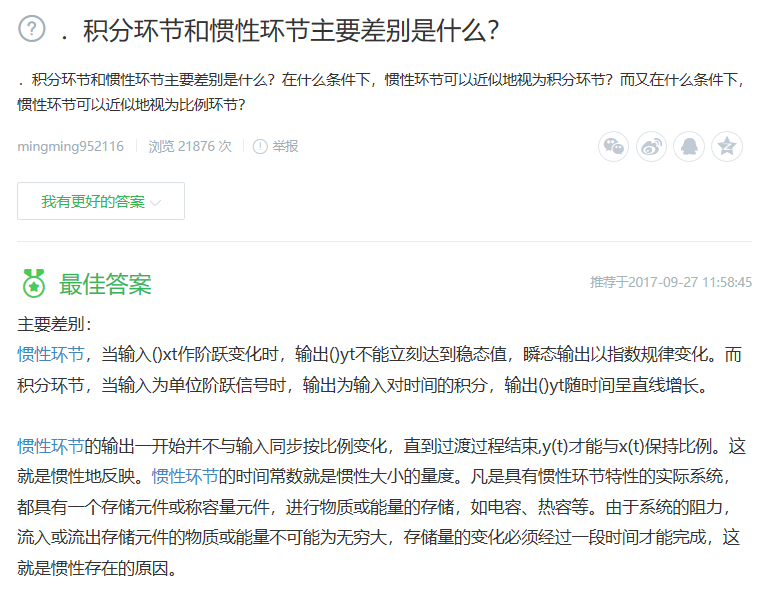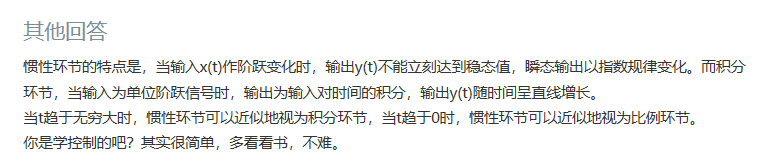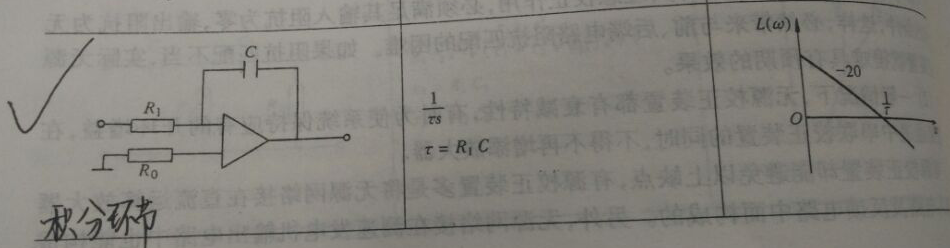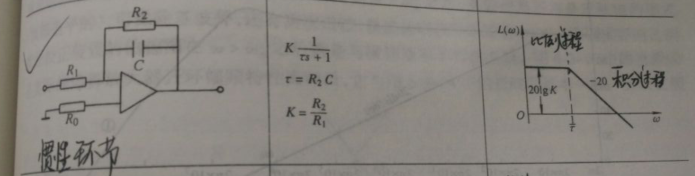可以看到，惯性环节的bode图分为两个部分，首先是比例过程，等过渡过程结束之后就是一个积分过程。

展开全文• ## MATLAB 传递函数的相关函数

千次阅读 多人点赞 2019-06-17 23:15:57
1. 连续时间传递函数 1.1 多项式形式传递函数 num = 1; % 分子多项式的系数 den = [2, 3]; % 分母多项式的系数 G = tf(num, den) % 求传递函数 在matlab中，运行上述代码，可以得到传递函数 1.2 因式乘积形式...
1. 连续时间传递函数

1.1 多项式形式传递函数

num = 1;             % 分子多项式的系数
den = [2, 3];        % 分母多项式的系数
G = tf(num, den)     % 求传递函数

在matlab中，运行上述代码，可以得到传递函数

1.2 因式乘积形式（零极点）传递函数

z = ;                % 零点
p = [2,3];              % 极点
k = -4;                 % 增益
G = zpk(z, p, k)        % 求传递函数

在matlab中，运行上述代码，可以得到传递函数

1.3 由状态空间描述得到传递函数（单输入单输出）

A = [0 1; -1 -1];                   % 系统矩阵
B = [0; 1];                         % 输入矩阵
C = [1 0];                          % 输出矩阵
D = 0;                              % 直接传递矩阵
[num, den] = ss2tf(A, B, C, D);     % 求分子多项式与分母多项式
G = tf(num, den)                    % 求传递函数   

在matlab中，运行上述代码，可以得到传递函数

2. 离散传递函数

如果想要从某个连续传递函数得到在特定采样周期下的离散函数，请看第3节。

离散时间传递函数的建立与连续时间基本一致，只需要引入一个采样周期。

请注意z变换与拉氏变换之间的区别，二者基本无共同点。

2.1 多项式形式离散传递函数

num = 1;             % 分子多项式的系数
den = [2, 3];        % 分母多项式的系数
T   = 1;             % 采样周期（1s）
G = tf(num, den, T)  % 求传递函数

在matlab中，运行上述代码，可以得到传递函数，采样时间为1s。

2.2 零极点形式离散传递函数

z = ;                % 零点
p = [2,3];              % 极点
k = -4;                 % 增益
T = 1;                  % 采样周期（1s）
G = zpk(z, p, k, T)     % 求传递函数

在matlab中，运行上述代码，可以得到传递函数，采样时间为1s。

3. 传递函数的化简与转化

3.1 连续传递函数与离散传递函数间的转化

3.1.1 连续传递函数到离散传递函数

已知一个连续传递函数。

运行下方代码，可以获得其在采样时间时，离散传递函数。

（其余形式的连续传递函数同理，均是使用函数c2d）

num = 1;             % 分子多项式的系数
den = [1, 1];        % 分母多项式的系数
G = tf(num, den)     % 连续传递函数
T = 1;               % 采样周期（1s）
Gz= c2d(G, T)        % 离散传递函数

3.1.2 离散传递函数到连续时间函数

已知一个离散传递函数，采样时间为。

运行下方代码，可以获取其连续传递函数。

（其余形式的离散传递函数同理，均是使用函数d2c）

num = 0.6321;           % 分子多项式的系数
den = [1, -0.3679];     % 分母多项式的系数
T   = 1;                % 采样周期（1s）
Gz = tf(num, den, T)    % 离散传递函数
Gs = d2c(Gz)            % 连续传递函数

3.2 因式乘积形式（零极点形式）与多项式形式的互相转化

3.2.1 因式乘积形式（零极点形式） 转化到 多项式形式

传递函数的变化：

z = ;                            % 零点
p = [2,3];                          % 极点
k = -4;                             % 增益
[num, den] = zp2tf(z, p, k);        % 因式乘积形式（零极点形式） 转化到 多项式形式
G = tf(num, den)                    % 多项式形式

3.2.2 多项式形式 转化到 因式乘积形式（零极点形式）

传递函数的变化：

num = [1,2];                        % 分子多项式的系数
den = [1,4,3];                      % 分母多项式的系数
[z, p, k] = tf2zp(num, den);        % 多项式形式 转化到 因式乘积形式（零极点形式）
G = zpk(z, p, k)                    % 零极点形式

3.3 传递函数的化简

传递函数的变化：

num = [1, 0];           % 分子多项式的系数
den = [1, 1, 0];        % 分母多项式的系数
G1 = tf(num, den)       % 带有可以对消项的传递函数
G2 = minreal(G1)        % 化简之后

3.4 多项式形式中首一形式与尾一形式的互相转化

3.4.1 将传递函数化简为首1形式

传递函数的变化：

num = 1;                % 分子多项式的系数
den = [10, 1];          % 分母多项式的系数
G1 = tf(num, den)       % 传递函数
G2 = minreal(G1)        % 化简为首一形式

3.4.2 将传递函数化简为尾1形式

传递函数的变化：

G   = tf(1, [1, 10]);               % 获取传递函数
num = cell2mat(G.Numerator);        % 分子多项式的系数
den = cell2mat(G.Denominator);      % 分母多项式的系数
num = num/den(end);                 % 除以分母最后一项
den = den/den(end);                 % 除以分母最后一项
G2  = tf(num, den)                  % 化简为尾一形式

3.5 求阶跃响应

Gs = tf(1, [1, 1]);         % 连续时间传递函数
Gz = c2d(Gs, 1);            % 获取对应的离散时间传递函数，采样时间T = 1s
step(Gs);                   % 连续传递函数的阶跃响应
hold on;                    % 保持图形
step(Gz);                   % 离散传递函数的阶跃响应
legend('阶跃响应-连续传递函数', '阶跃响应-离散传递函数'); % 添加图例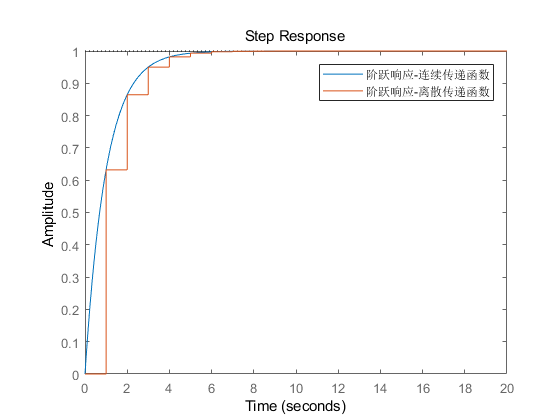展开全文MATLAB 自动控制原理 现代控制理论 线性系统理论
• 给定传递函数的零点，极点以及增益K求原传递函数
tf2zp命令
前几期博客中有涉及到这个命令，这个可以根据传递函数的分子分母系数来求得传递函数的零点，极点以及增益K
zp2tf
相反，通过这个命令可以根据零点，极点，增益K求得原传递函数
EXAMPLE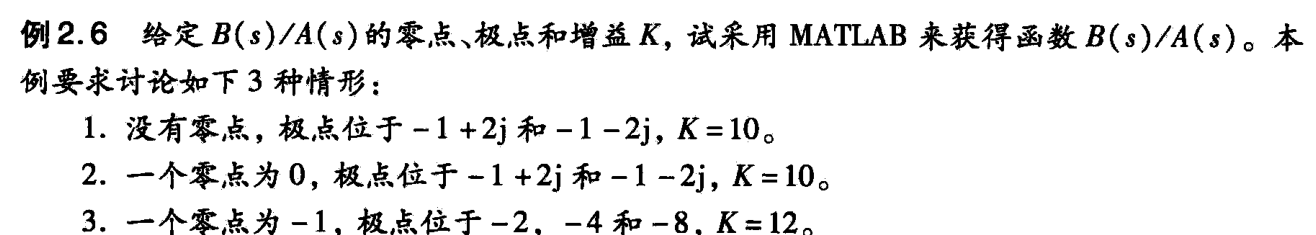1
>> z = [];
>> p = [-1+2*i;-1-2*i];
>> K = 10;
>> [num,den] = zp2tf(z,p,K)

num =

0     0    10

den =

1     2     5

>> printsys(num,den,'s')

num/den =

10
-------------
s^2 + 2 s + 5


2
>> z = ;
>> p = [-1+2*i;-1-2*i];
>> K = 10;
>> [num,den] = zp2tf(z,p,K)

num =

0    10     0

den =

1     2     5

>> printsys(num,den,'s')

num/den =

10 s
-------------
s^2 + 2 s + 5


3
>> z = -1;
>> p = [-2;-4;-8];
>> K = 12;
>> [num,den] = zp2tf(z,p,K)

num =

0     0    12    12

den =

1    14    56    64

>> printsys(num,den,'s')

num/den =

12 s + 12
------------------------
s^3 + 14 s^2 + 56 s + 64


Notes
需要注意的是，我们在输入零点以及极点的时候，每个要以分号分隔，也就是说，每一个解表示一列。


展开全文• 自动控制原理（4）——传递函数、典型环节的传递函数 微分方程模型 优点：是时间域的数学模型，比较直观，它用时间域的方式，描述系统输入和输出变量之间的关系 ​ 在给定初始条件和输入信号后，借助计算机可以...
自动控制原理（4）——传递函数、典型环节的传递函数
微分方程模型

优点：是时间域的数学模型，比较直观，它用时间域的方式，描述系统输入和输出变量之间的关系
​           在给定初始条件和输入信号后，借助计算机可以迅速而准确地求出输出响应

缺点：不便于分析结构或参数变化对系统性能的影响

微分方程的方法研究控制系统对于参数变化或结构形式的改变的分析具有局限性
一、传递函数

复数域的数学模型

在研究系统结构或参数变化对性能的影响方面非常方便

定义：线性定常系统的传递函数是零初始条件下系统输出量的拉氏变换与系统输入量的拉氏变换之比

传递函数针对线性定常系统定义，对非线性的、或时变的系统是不适用的

零初始条件

系统的输入在时间t>0时才作用于系统，在零时刻之前，系统的输入及其各阶导数均为零
输入在施加于系统之前，系统为稳态，也就是说，在零时刻之前，系统输出及其各阶导数均为零

性质

传递函数反映系统自身固有特性，与输入和初始条件无关

传递函数与微分方程：将微分方程运算符d/dt用复数s置换可以得到传递函数，反之亦然

不同的物理系统可能有相同的传递函数（如相似系统），而同一系统可以有不同的传递函数

一个传递函数只能表示一个输入对一个输出的函数关系，如果是多输入多输出系统，可以用传递函数矩       		  阵表示

传递函数与单位脉冲响应之间是拉氏变换与拉氏反变换的关系

一般情况下，传递函数分子的阶数m与分母的阶数n满足n≥m（称为物理现实性条件）

局限性

只适于线性定常系统的表达
不反映初始状态的信息
不反映系统内部的信息

二、典型环节的传递函数
构成线性定常控制系统的七个环节：比例环节，微分环节，一阶微分环节，二阶微分环节，积分环节，惯性环节，振荡环节
1、比例环节：c(t)=Kr(t)   G(s)=K

K为比例系数
比例环节又称无惯性环节或放大环节
比例环节既无零点，又无极点
性质：比例环节输出与输入成正比，不失真也不滞后
实例：理想的杠杆、放大器、测速发电机、电位器等

2、惯性环节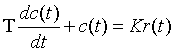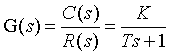T为时间常数
K为放大系数（比例系数）
惯性环节无零点
性质：当系统输入时单位阶跃信号时系统输出按单调指数规律上升

3、积分环节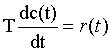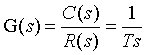T为积分时间常数
积分环节无零点
当输入是单位阶跃信号时，积分环节的输出以固定斜率1/T单调上升

4、振荡环节ωn为无阻尼自然振荡频率

ζ为阻尼比

5、微分环节

理想微分环节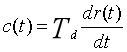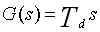微分环节的输出与输入的一阶导数成正比，因此微分环节能预示输入信号的变化趋势，常用来改善控制系统的动态性能

6、一阶微分环节

理想一阶微分环节（比例微分环节）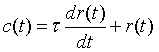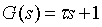τ为时间常数
比例微分环节可以抑制震荡，提高控制系统的稳定性，改善系统的动态性能

7、二阶微分环节

理想二阶微分环节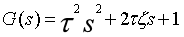τ为时间常数
ζ为阻尼比
二阶微分环节没有极点，有2个零点
实际中也不存在真正的二阶微分环节

8、延滞环节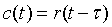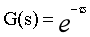τ叫做延滞时间，又称死区时间
具有延滞环节的系统叫做延滞系统
性质：延滞环节将输入延迟τ时间后才输出。系统中存在延滞环节时，对系统的稳定性不利


展开全文• ## 复阻抗法 求解 传递函数

万次阅读 多人点赞 2018-11-26 19:24:15
1、复阻抗法求传递函数 电阻的传递函数 ：RRR 电容的传递函数 ： 1CS\frac 1{CS}CS1​ 电感的传递函数 ： LSLSLS 2、并联电路 在此之所以没有写串联电路，是因为串联电路太简单了，就是简单的复阻抗想加即可。并联...
• ## 传递函数推导

千次阅读 2018-03-14 11:04:53
• 开环传递函数的概念是针对闭环控制系统来说的，而不是指开环控制系统的传递函数 开环传递函数为闭环系统人为地断开主反馈通路所得到的传递函数
• 数据库部分函数依赖 完全函数依赖 传递函数依赖 第一范式、第二范式、第三范式、BCNF范式区别 在理解函数依赖之前，先来看一下函数依赖分析： 在关系中，包括在任何候选码中的属性称为主属性；不包括在任何候选...部分函数依赖 完全函数依赖 数据库范式
• ## 复阻抗传递函数

千次阅读 2020-07-03 21:38:35
在画控制系统方框图时，需要将从输入量到输出量的所有中间变量及传递函数写出，以便画图，在电路系统中时常遇到电感和电容，其传递函数推导如下： 1.电容传递函数： 电容输入输出关系 以uc(t)u_c(t)uc​(t)为输出...
• 1 传递函数作为参数 function hello(){ console.log("hello"); } function say(func){ func(); } say(hello); 注意：say(hello)不能写成say(hello())，后者会直接调用hello()函数并返回一个undefined...js javascript setTimeout
• ## MATLAB实现传递函数

万次阅读 多人点赞 2019-01-22 17:50:51
1、简单的传递函数模型   num=[1,10]； den=[1,5,4,3,2]; G=tf(num,den)   2、零极点模型 KGain=K; %系统增益K Z=[1; 2; 3]； %零点 P=[4; 5; 6]; %极点 G=zpk(Z,P,KGain) 3、反馈系统 G1=tf(3,[1,...
•matlab
• ## 传递函数零极点

千次阅读 2020-03-05 23:19:05
传递函数零极点1. 概念2. 利用零极点分析系统 1. 概念 系统传递函数经过因式分解可写为： G(s)=Y(s)R(s)=(s−z1)(s−z2)⋯(s−zj)(s−zm)(s−p1)(s−p2)⋯(s−pi)(s−pn)k1 G(s)=\frac{Y(s)}{R(s)}=\frac{(s-z_{1})...经验分享
• 一、RC电路的微分方程与传递函数 根据电路关系可得：Ur=I*R+Uc，I=dUc/dt 在零初始条件下，对方程组两边同时做拉氏变换得 其传递函数为： 令R*C=T， 1/(T*S+1)是典型的惯性环节 二、LRC电路的微分方程与...抽象代数 算法
• ## vue props 传递函数

千次阅读 2020-04-30 20:27:06
通过 props 传递 函数 看看有啥用。 // 父组件 </template> <div> <children :add='childrenClick'></children> <p>{{countF}}</p> </div> </template>...
• 侵删 BP网络常用传递函数: BP网络的传递函数有多种。Log-sigmoid型函数的输入值可取任意值，输出值在0和1之间；tan-sigmod型传递函数tansig的输入值可取任意值，...线性传递函数purelin的输入与输出值可取任意BP网络
• 模型准备：二阶传递函数模型1.1 二阶传递函数模型1.1.1 时域模型1.1.2 频域模型1.2 二阶传递函数公式和参数2. 开始建模：在simulink中搭建二阶传递函数模型2.1 从simulink库中添加传递函数模块2.2 设置传递函数参数...matlab simulink
• 传递函数框图 r:期望输入 u： 控制器输入 y： 期望输出 d： 扰动输入 K：控制器 G: 系统模型 灵敏度函数 S=(I+GK)−1S =(I+GK)^{-1}S=(I+GK)−1,是扰动d到期望输出y的闭环传递函数，设计控制系统使得S越小越好 回路...
• MTF是Modulation Transfer Function的英文简称，中文为调制传递函数。 MTF是指调制度随空间频率变化的函数称为调制度传递函数。最开始是为了说明镜头的能力。在各个摄像头镜头中经常采用MTF描述镜头的MTF曲线，表明...
• 1、构造描述函数1.1 传递函数（1） Matlab可以这样构造上式num=[10 30 20]; den=[1 12 47 60]; sysc=tf(num,den) 运行结果（2） 根据零极点构造表达式：z=[-1 -2]; p=[-3 -4 -5]; k=10; sysc=zpk(z,p,k) 运行...自控原理 Matlab
• 最近在做毕业设计，自己的控制对象描述形式是传递函数，而书里看到的大部份被控对象都是差分方程的形式，就想有没有这种转换的方法，网上搜索了一圈，总结如下。 步骤一，先建立传递函数方程 首先给定一个连续的传递...MATLAB 差分方程
•    传递函数与状态空间之间可相互转换，可以使用的matlab函数有 [A,B,C,D] = tf2ss(NUM,DEN) [NUM,DEN] = ss2tf(A,B,C,D,iu)    传递函数的形式唯一，但状态空间的形式不唯一，可以有多种。 1、一阶惯性...matlab 状态空间 simulink
• 1：传递函数的几种Matlab输入方式 1.1、显示多项式的传递函数 matlab语句：分子分母的各项式系数按照降次排序分别计入num=[ ]，den=[ ]中，缺项系数补零。 传递函数 sys=tf(num,den) 1.2、因子形式的传递函数 ...
• 传递函数波特图的画法和理解传递函数波特图定义如何根据传递函数画波特图相角裕度的理解传递函数波特图的理解 传递函数波特图定义 首先波特图是描述系统频率和增益或者相角关系的坐标图，横坐标一般是ω(rad/s)或者...
• 传递函数的生成： 可自行百度 这里只讲feedback函数的使用: feedback可用于传递函数求解，比如单位负反馈系统，前向传递函数为，求闭环传递函数。 此时我想说明的是当g是一个抽象的传递函数，意思是你不...
• 数据库，部分函数依赖，传递函数依赖，完全函数依赖，三种范式的区别数据库 范式
• ## PID控制器的传递函数推导

万次阅读 多人点赞 2018-07-07 17:43:09
传递函数的定义: 传递函数是指零初始条件下线性系统响应（即输出）量的拉普拉斯变换（或z变换）与激励（即输入）量的拉普拉斯变换之比。记作G(s)=Y(s)U(s)G(s)=Y(s)U(s)G_{(s)}=\frac{Y_{(s)}}{U_{(s)}}，其中Y(s)Y...
• 前言：我们在Matlab/Simulink做实验仿真的对象一般习惯使用传递函数，但是真正转化到单片机中时，又无法识别传递函数，因此需要转化为差分方程来处理。最近遇到转化差分方程的问题，写一个博客记录。 一、传递函数...matlab 算法
• MATLAB建立传递函数的方法 传递函数 TF对象构造法 num =[1 5]; den=[1 16 51 36]; G=tf(num,den); conv命令法 num=[1 5]; den=conv([1,1],conv([1,3],[1...MATLAB...